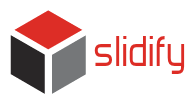# Widgets

## Slidify Workshop

Ramnath Vaidyanathan

A widget is a collection of stylesheets, javascripts and layouts that add additional functionality to a slide deck.

## MathJax

---
title: MathJax
widgets: [mathjax]
mode: draft
---

## Mathjax ##

\begin{aligned} \nabla \times \vec{\mathbf{B}} -\, \frac1c\, \frac{\partial\vec{\mathbf{E}}}{\partial t} & = \frac{4\pi}{c}\vec{\mathbf{j}} \\ \nabla \cdot \vec{\mathbf{E}} & = 4 \pi \rho \\ \nabla \times \vec{\mathbf{E}}\, +\, \frac1c\, \frac{\partial\vec{\mathbf{B}}}{\partial t} & = \vec{\mathbf{0}} \\ \nabla \cdot \vec{\mathbf{B}} & = 0 \end{aligned}


## Try It

1. Open demos/widgets/mathjax/index.Rmd
2. Slidify and Open index.html

You can experiment by adding other equations.

## Quiz

---
title: Interactive Quiz
widgets: [bootstrap, quiz]
mode: draft

## Question 1

What is 1 + 1?

1. 1
2. _2_
3. 3

*** .hint

This is a hint

*** .explanation

This is an explanation


## Try It

1. Open demos/widgets/quiz/index.Rmd
2. Slidify and Open index.html
You can find more question types here. Press p on any slide to get the Rmd source, paste it in your index.Rmd file and see how it works.